# ML Aggarwal Class 8 Solutions for ICSE Maths Chapter 10 Algebraic Expressions and Identities Objective Type Questions

## ML Aggarwal Class 8 Solutions for ICSE Maths Chapter 10 Algebraic Expressions and Identities Objective Type Questions

Mental Maths
Question 1.
Fill in the blanks:
(i) A symbol which has a fixed value is called a ……….
(ii) A symbol which can be given various numerical values is called ……….. or ………
(iii) The various parts of an algebraic expression separated by + or – sign are called ………..
(iv) An algebraic expression having ………… terms is called a binomial.
(v) Each of the quantity (constant or literal) multiplied together to form a product is called a ………… of the product.
(vi) The terms having the same literal coefficients are called a ………… otherwise they are called …………
(vii) Degree of the polynomial is the greatest sum of the powers of ………… in each term.
(viii)An identity is equality which is true for ………… of variables in it.
(ix) (a + b)2 = …………
(x) (x + a) (x + b) = x2 + ………… + ab.
(xi) Dividend = ………… + remainder.
Solution:Question 2.
State whether the following statements are true (T) or false (F):
(i) An algebraic expression having only one term is called a monomial.
(ii) A symbol which has fixed value is called a literal.
(iii) The term of an algebraic expression having no literal factor is called its constant term,
(iv) In any term of an algebraic expression the constant part is called literal coefficient of the term.
(v) An algebraic expression is called polynomial if the powers of the variables involved in it in each term are non-negative integers.
(vi) 5x2y2z, 3x2zy2, – $$\frac{4}{5}$$ zx2y2 are unlike terms.
(vii) 5x + $$\frac{2}{x}$$ + 7 is a polynomial of degree 1.
(viii) 3x2y + 7x + 8y + 9 is a polynomial of degree 3.
(ix) Numerical coefficient of -7x3y is 7.
(x) An equation is true for all values of variables in it.
(xi) (a – b)2 + 2ab = a2 + b2.
Solution: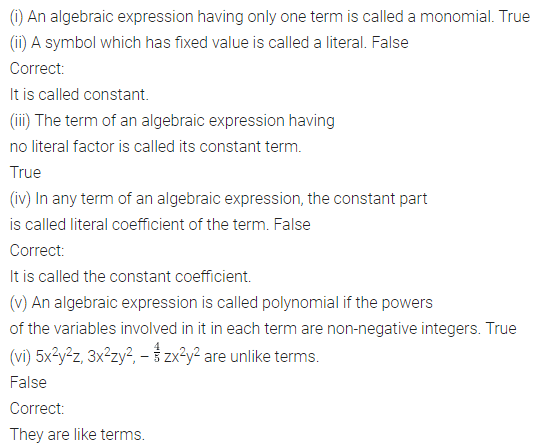Multiple Choice Questions
Choose the correct answer from the given four options (3 to 18):
Question 3.
The literal coefficient of -9xyz2 is
(a) -9
(b) xy
(c) xyz2
(d) -9xy
Solution: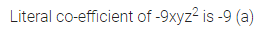Question 4.
Which of the following algebraic expression is
(a) 3x2 – 2x + 7
(b) $$\frac{5 x^{3}}{2 x}$$ + 3x2 + 8
(c) 3x + $$\frac{2}{x}$$ + 7
(d) $$\sqrt{2}$$x2 + $$\sqrt{3}$$x + $$\sqrt{6}$$
Solution:Question 5.
Which of the following algebraic expressions is not a monomial?
(a) 3x × y × z
(b) -5pq
(c) 8m2 × n ÷ 31
(d) 7x ÷ y – z
Solution: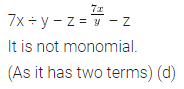Question 6.
Degree of the polynomial 7x2yz2 + 6x3y2z2 – 5x + 8y is
(a) 4
(b) 5
(c) 6
(d) 7
Solution:Question 7.
a(b -c) + b(c – a) + c(a – b) is equal to
(a) ab + bc + ca
(b) 0
(c) 2(ab + bc + ca)
(d) none of these
Solution:Question 8.
$$\frac{7}{5}$$xy – $$\frac{2}{3}$$xy + $$\frac{8}{9}$$ xy is equal toSolution: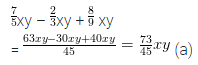Question 9.
(3p2qr3) × (-4p3q2r2) x (7pq3r) is equal to
(a) 84p6q6r6
(b) -84p6q6r6
(c) 84p6q5r6
(d) -84p6q5r6
Solution:Question 10.
3m × (2m2 – 5mn + 4n2) is equal to
(a) 6m3 + 15m2n – 12mn2
(b) 6m3 – 15m2n + 12mn2
(c) 6m3 – 15m2n – 12mn2
(d) 6m3 + 15m2n + 12mn2
Solution:Question 11.
Volume of a rectangular box whose adjacent edges are 3x2y, 4y2z and 5z2x respectively is
(a) 60xyz
(b) 60x2y2z2
(c) 60x3y3z3
(d) none of these
Solution:Question 12.
(x – 1) (x + 2) is equal to
(a) 2x + 3
(b) x2 + 2x + 2
(c) x2 + 3x + 2
(d) x2 + 2x + 3
Solution:Question 13.
If x + $$\frac{1}{x}$$ = 2, then x2 + $$\frac{1}{x^{2}}$$ is equal to
(a) 4
(b) 2
(c) 0
(d) none of these
Solution:Question 14.
If x2 + y2 = 9 and xy = 8, then x + y is equal to
(a) 25
(b) 5
(c) -5
(d) ±5
Solution: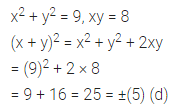Question 15.
(102)2 – (98)2 is equal to
(a) 200
(b) 400
(c) 600
(d) 800
Solution: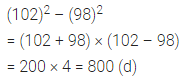Question 16.
-50x3y2z2 divided by -5xyz is equal to
(a) 10xyz
(b) 10x2yz
(c) -10xyz
(d) -10x2yz
Solution:Question 17.
96 × 104 is equal to
(a) 9984
(b) 9974
(c) 9964
(d) none of these
Solution: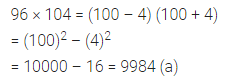Question 18.
If the area of a rectangle is 24(x2yz + xy2z + xyz2) and its length is 8xyz, then its breadth is
(a) 3(x + y + z)
(b) 3xyz
(c) 3(x + y – z)
(d) none of these
Solution: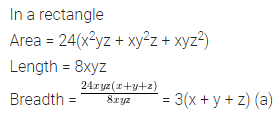Higher Order Thinking Skills (Hots)
Question 1.
Using the identity (a + b) = a2 + 2ab + b2, derive the formula for (a + b + c)2. Hence, find the value of (2x – 3y + 4z)2.
Solution:Question 2.
Using the product of algebraic expressions, find the formulas for (a + b)2 and (a – b).
Solution: